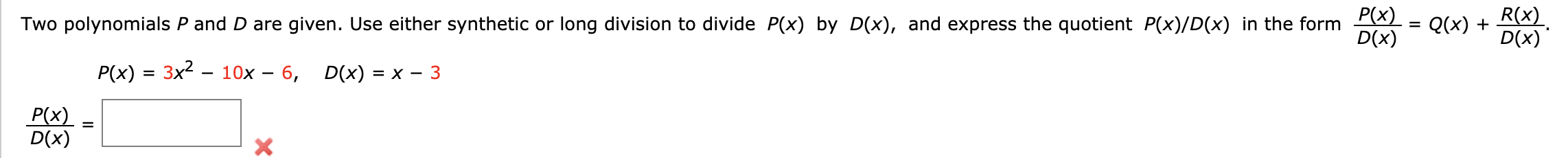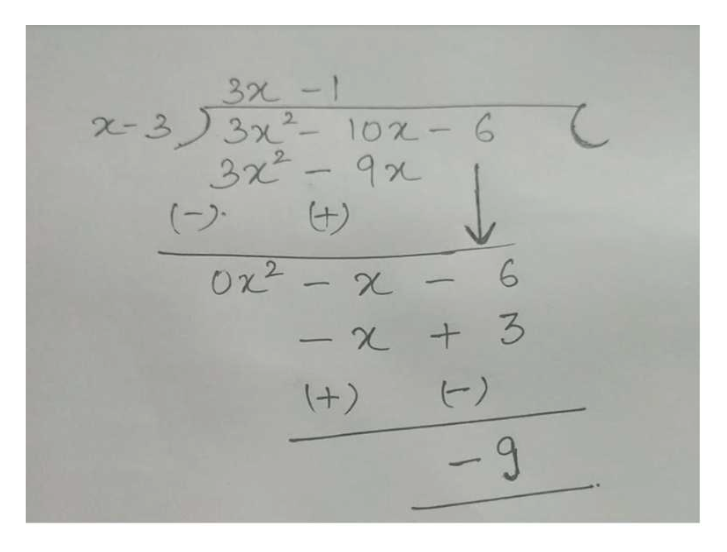# P(x)D(x)R(x)D(x)Two polynomials P and D are given. Use either synthetic or long division to divide P(x) by D(x), and express the quotient P(x)/D(x) in the formQ(x)=10x 6,P(x) 3x2D(x)= X 3P(x)D(x)

Question
12 viewshelp_outlineImage TranscriptioncloseP(x) D(x) R(x) D(x) Two polynomials P and D are given. Use either synthetic or long division to divide P(x) by D(x), and express the quotient P(x)/D(x) in the form Q(x) = 10x 6, P(x) 3x2 D(x) = X 3 P(x) D(x) fullscreen
check_circle

Step 1

According to the given information:

Step 2

It is required to find the remainder and qu...help_outlineImage Transcriptionclose3 -1 2-3 3x- 102-6 3x2 (> 9x Ox2 6 - t3 (+) - 9 fullscreen

### Want to see the full answer?

See Solution

#### Want to see this answer and more?

Solutions are written by subject experts who are available 24/7. Questions are typically answered within 1 hour.*

See Solution
*Response times may vary by subject and question.
Tagged in

### Other